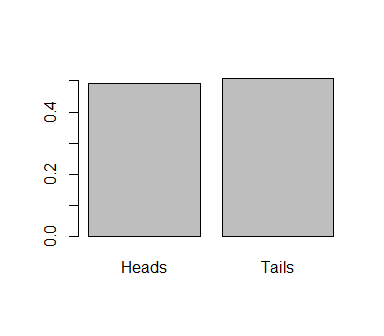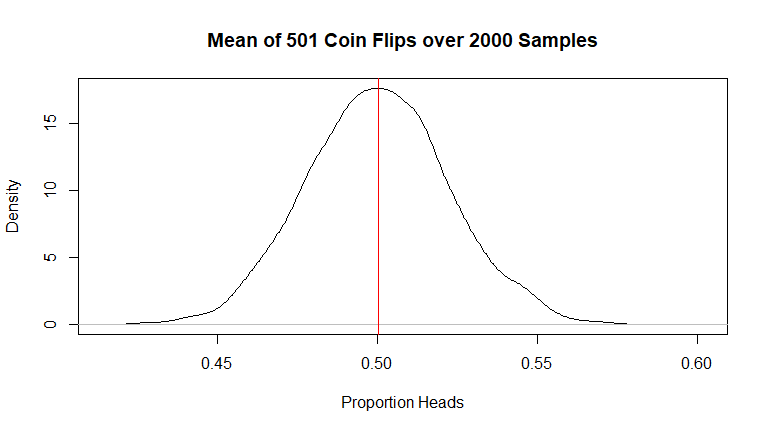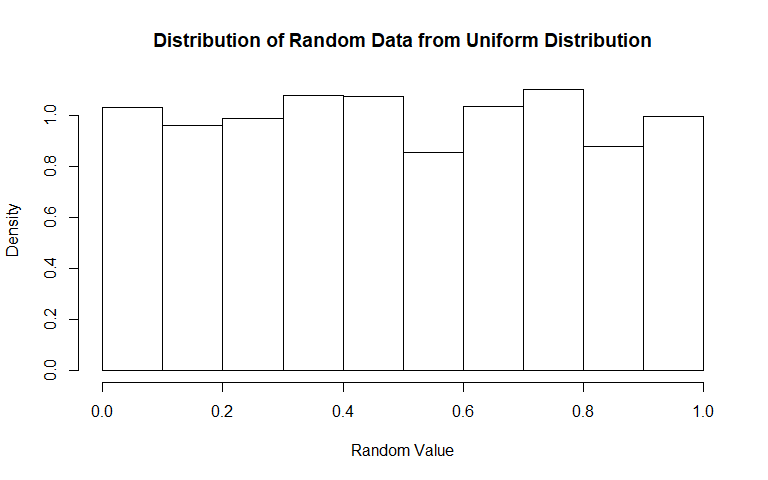# Lecture 11: Simulating Data

## Recap

• Up to now, we’ve been looking at how to manipulate objects in R
• And how to analyze data in R
• Looking at the distributions of those variables
• As well as how two variables relate to each other - dependence, correlation, explanation

## And now!

• What we’ll be moving into soon is how to use these tools so that we can take data and actually learn things
• Today we’re going to get ready for that
• How?
• We’re going to learn some fake things
• Via simulation, i.e. creating fake data

## Simulation

• Why do this? Why not just use real data?
• Because with real data, we don’t know what the right answer is
• So if we do some method, and it gives us an answer, how do we know we did the method right?
• Simulation lets us know the right answer so we can check whether or not we get that right answer
• And if we do, we know the method works (at least in our fake scenario), so now we can apply it to some real data

## The purpose of data analysis

• When it comes down to it, what is the purpose of data analysis? Why do we work with data?
• When we work with data, we have this idea that there exists a true model
• The true model is the way the world actually works!
• But we don’t know what that true model is

## The purpose of data analysis

• So that’s where the data comes in
• The true model is what generated the data (the ‘data generating process’)
• So by looking at the data, and incorporating what we think we know about the true model, we’re trying to work backwards to figure out what it is that generated that data - and that’s the true model!
• With simulation, we know what generated the data and what the true model is. So we know the right answer and can check how close we get.

## Example

• We’ve covered this example before
• Let’s generate 501 coin flips
• The true model will be that this will generate heads half the time and tails half the time
``coins <- sample(c("Heads","Tails"),501,replace=T)``

## Example

• Now let’s take that data as given and analyze it in our standard way!
• The proportion of heads is `mean(coins=='Heads') =`0.493014
• And we can look at the distribution, as we would:
``barplot(prop.table(table(coins)))``## Example

• So what’s our conclusion?
• We’d come to the conclusion that the true model generates heads 0.493014 of the time
• .500 is correct, so pretty close! But not exact. Did this whole thing work or not?
• What if it always errs on the same side? Then it’s not a good method at all!

## Simulation in a Loop

• We can go a step further by doing this simulation over and over again in a loop!
• This will let us tell whether our method gets it right on average
• And, when it’s wrong, how wrong it is!

## Simulation in a Loop

``````#A blank vector to hold our results

#Let's run this simulation 2000 times
for (i in 1:2000) {
#Re-create data using the true model
#Re-perform our analysis
#And store the result
}

#Let's see what we get on average
``````##
## ============================================================
## Statistic   N   Mean  St. Dev.  Min  Pctl(25) Pctl(75)  Max
## ------------------------------------------------------------
## propHeads 2,000 0.500  0.022   0.427  0.485    0.515   0.589
## ------------------------------------------------------------``````

## Simulation in a Loop

``````#And let's look at the distribution of our findings## Simulation in a Loop

• Now that’s pretty exact!
• What are we learning here?
• The method that we used (simply taking the proportion of heads) will, on average, give us the right answer (.5). Good! Now, if we’re interested in a similar case in the real world, we can apply this method.
• Keeping in mind that in any given sample that we actually observe, it might be a little off.

## Now Imagine

• Imagine we didn’t know the answer was .5. What would have happened?
• Wonder “what proportion of the time will a coin be heads?” (what is the true model?)
• Collect data on coin flips
• Perform our analysis method - take proportion of heads, and get 0.493014
• Come to the conclusion that the true model is that the coin produces heads 0.493014 of the time.
• We wouldn’t be dead on, but on average we’d be right!

## Generating Other Kinds of Data

• We can use `sample()` as before to pick random data from different categories like Heads and Tails, or integers (say, `1:10`)
• R also has a whole buncha functions to generate random data from other kinds of distributions. See `help(Distributions)`.
• We will focus on two:
• The uniform distribution assigns equal probablity to each value in its range (default 0 to 1)
• The normal distribution is a bell curve - observations near the mean are very common, observations far away from the mean very rare

## Generating uniform data

• `runif(thismanyobs,min,max)` will draw `thismanyobs` observations from the range of `min` to `max`.
• `runif(thismanyobs)` will assume `min=0` and `max=1`
``````uniformdata <- runif(5)
uniformdata``````
``##  0.1267818 0.8207126 0.2619525 0.4291249 0.1961915``

## Generating Uniform Data

``````uniformdata <- runif(2000)
hist(uniformdata,xlab="Random Value",main="Distribution of Random Data from Uniform Distribution",probability=TRUE)``````## Generating Uniform Data

• Good for variables that should be bounded - “percent male” can only be 0-1
• Gives even probability of getting each value

## Generating Normal data

• `rnorm(thismanyobs,mean,sd)` will draw `thismanyobs` observations from a normal distribution with mean `mean` and standard deviation `sd`
• `rnorm(thismanyobs)` will assume `mean=0` and `sd=1`
``````normaldata <- rnorm(5)
normaldata``````
``##  -2.2990547  0.1119890 -0.3365734  2.2590853 -0.7877652``

## Generating Normal Data

``````normaldata <- rnorm(2000)
hist(normaldata,xlab="Random Value",main="Distribution of Random Data from Normal Distribution",probability=TRUE)``````